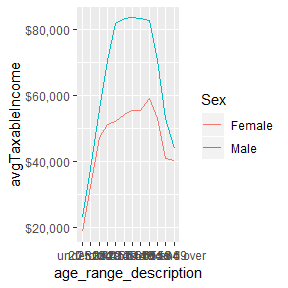# 2015-16 taxstats miscellany

#### 2019-03-23

library(data.table)
library(hutils)
library(magrittr)
library(ggplot2)

library(grattan)  # needs to be before require_taxstats..
require_taxstats()
require_taxstats1516()
s1516 <-
as.data.table(sample_file_1516_synth) %>%
setkey(Taxable_Income) %>%
mutate_ntile("Taxable_Income", n = 100L) %>%
mutate_ntile("Taxable_Income", n = 10L)
s1516[, tax := income_tax(Taxable_Income, "2015-16", .dots.ATO = .SD)]

## Negative gearing

### Tax benefits

s1516[, taxNoNG := income_tax(Taxable_Income - pminC(Net_rent_amt, 0),
fy.year = "2015-16",
.dots.ATO = .SD)]
s1516[, benefit_due_NG := taxNoNG - tax]

#### by decile

benefitsNG_by_Decile <-
s1516[, .(avgBenefitNG = mean(benefit_due_NG)), keyby = "Taxable_IncomeDecile"]

copy(benefitsNG_by_Decile) %>%
setnames("Taxable_IncomeDecile", "Taxable Income Decile") %>%
.[, Share := paste0(round(100 * avgBenefitNG / sum(avgBenefitNG)), "%")] %>%
.[, avgBenefitNG := round(avgBenefitNG, -2)] %>%
setnames("avgBenefitNG", "Average benefit from NG / ") %>% kable(align = "rrr") Taxable Income Decile Average benefit from NG / Share
1 0 2%
2 100 2%
3 100 3%
4 100 5%
5 200 6%
6 200 8%
7 200 9%
8 300 12%
9 500 17%
10 1000 36%

### Average taxable income, by age and gender, 2015

s1516[, .(avgTaxableIncome = mean(Taxable_Income)), keyby = .(age_range, Gender)] %>%
.[age_range_decoder, on = "age_range"] %>%
.[, Sex := if_else(Gender == 1, "Female", "Male")] %>%
ggplot(aes(x = age_range_description,
y = avgTaxableIncome,
group = Sex,
color = Sex)) +
geom_line() +
scale_y_continuous(labels = function(x) paste0("\$", prettyNum(x, big.mark = ",")))